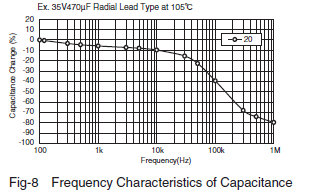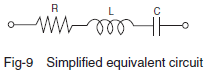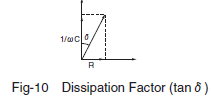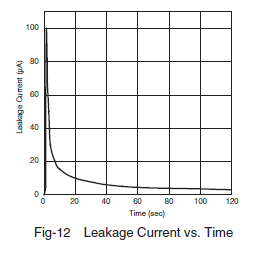### Aluminum electrolytic capacitor Basic Electrical Characteristics

Contents
Capacitance
tanδ(Dissipation Factor)
Leakage Current（LC)
##### Capacitance
The larger the surface area of an electrode is, the higher the capacitance (capacity for storing electricity) is. For aluminum electrolytic capacitors, the capacitance is measured under the standard measuring conditions of 20°C and a 120Hz AC signal of about 0.5V. Generally, as the temperature rises, the capacitance increases; as the temperature decreases, the capacitance decreases (Fig. 7). With a higher frequency, the capacitance is smaller; with a lower frequency, the capacitance is larger (Fig. 8).##### Tanδ( also called tangent of loss angle or dissipation factor)
(Fig. 9) is a simplified model of the equivalent circuit shown in (Fig. 2). For an ideal capacitor with an equivalent series resistance of R = 0, the tanδ shown in (Fig. 10) is zero. For an aluminum electrolytic capacitor, the equivalent series resistance (R) is not zero due to the presence of resistance of the electrolyte and paper separator and other contact resistances. 1/ωC and R are correlated as shown in (Fig. 10) and Equation (2).

ω：2πf
π＝Circular constant、f：Frequency（f ＝ 120Hz）##### Leakage Current (LC)
As a feature of an aluminum electrolytic capacitor, when DC voltage is applied to it, the oxide layer that acts as a dielectric in the electrolyte allows a small amount of electric current to flow in it. The small amount of current is called a leakage current (LC). An ideal capacitor does not allow the leakage current to flow (this is not the case for charging current).
The leakage current (LC) changes with time as shown in (Fig. 12). Note that LC, decreasing with time, will reach a steady-state value. Therefore, the specifications of LC are defined as a value measured several minutes after the beginning of the application of the rated voltage at 20°C. As the temperature rises, the LC increases; as the temperature decreases, the LC decreases (Fig.13). As the applied voltage decreases, the LC decreases